Project tutorial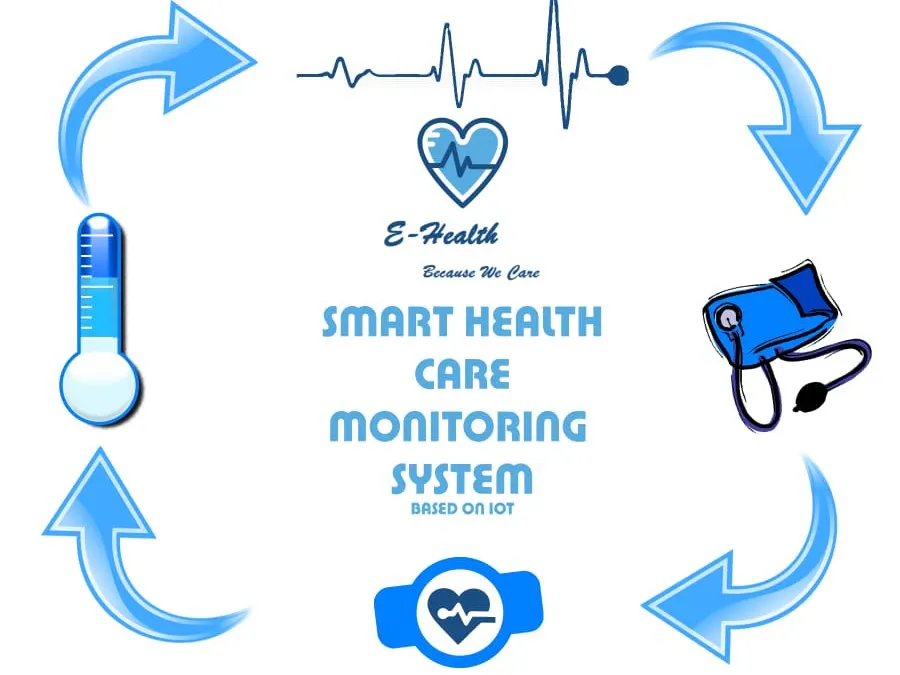# Smart Health Care Monitoring System Based on IoT © CC BY-NC-ND

This project includes hardware which will measure blood pressure, heart rate, ECG & temperature, and send to Android application & Firebase.

• 904 views
• 0 comments
• 10 respects

## Components and suppliesArduino UNO & Genuino UNO
×1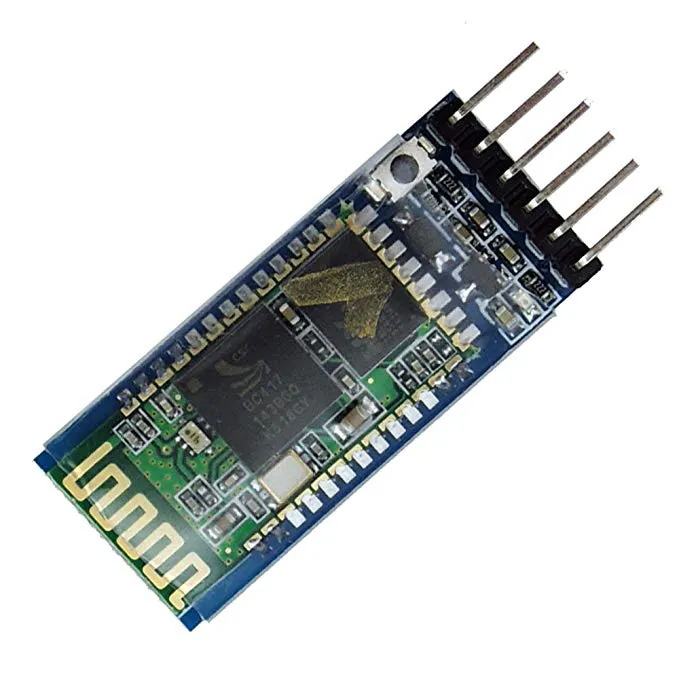HC-05 Bluetooth Module
×1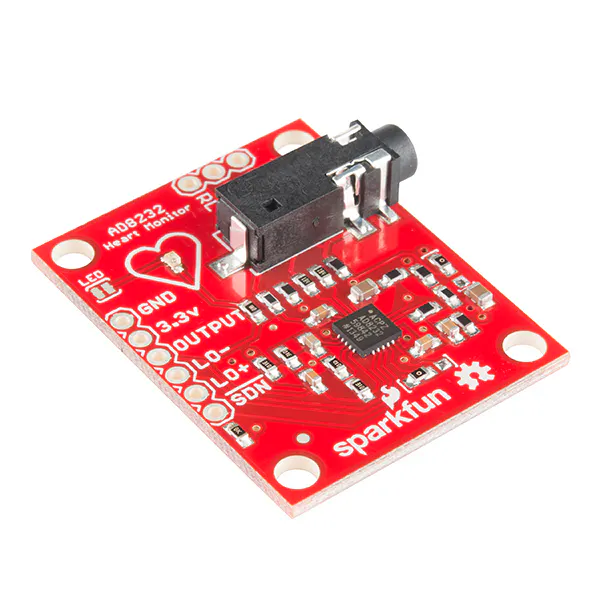SparkFun Single Lead Heart Rate Monitor - AD8232
×1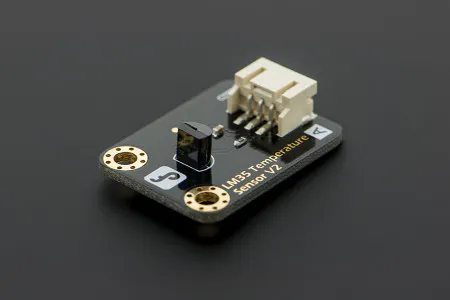DFRobot Gravity: Analog LM35 Temperature Sensor For Arduino
×1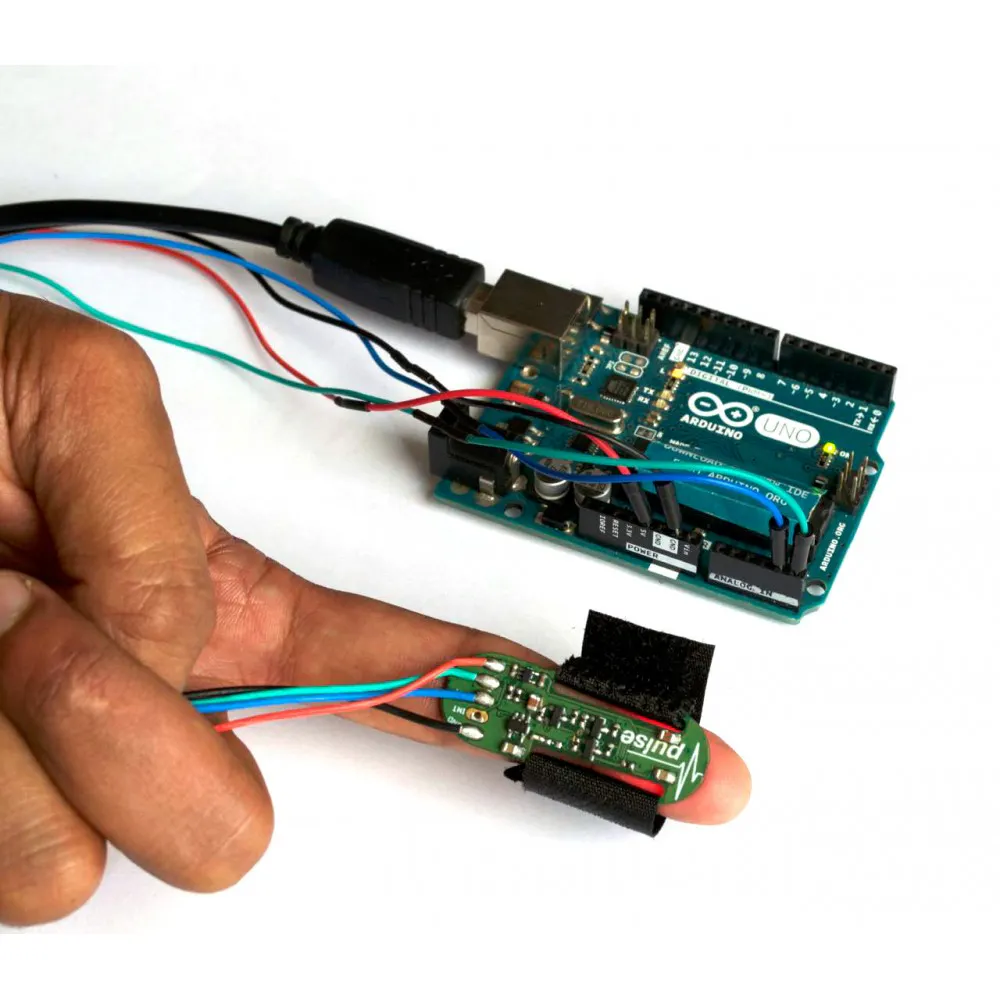ProtoCentral Pulse Oximeter & Heart Rate Sensor based on MAX30100
×1Breadboard (generic)
×1
 Sunrom Blood Preasure Module
×1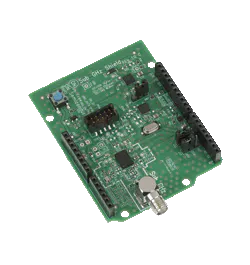On Semiconductor EU-SIGFOX-GEVB
×1

## About this project

### Introduction

This project is significant in various ways because in today's world, everyday many lives are affected because the patients are not timely and properly operated. Also for real time parameter values are not efficiently measured in clinic as well as in hospitals.Sometimes it becomes difficult for hospitals to frequently check patient’s conditions. Also continuous monitoring of ICU patients is very difficult. To deal with these types of situations, our system is beneficial. Our system is designed to be used in hospitals and homes also for measuring and monitoring various parameters like temperature, ECG, heart rate, blood pressure. The results can be recorded using Arduino. Also the doctors can see those results on android app. The system will also generate an alert notification which will be sent to doctor. Our system is useful for monitoring health system of every person through easily attach the device and record it. In which we can analysis patient’s condition through their past data, we will recommend medicines if any emergency occurred through symbolic A.I.

### Work Flow:

First of all we have to make Communication between Arduino and android app using Bluetooth. for the android code for this communication kindly follow the link below

https://solderer.tv/data-transfer-between-android-and-arduino-via-bluetooth/

now after establishing the communication successfully start creating android app using android studio and use the code from above link after this send some data to arduino in our scenario we have 4 module so we have 16 combination like patient want only temperature data or ecg, Blood pressure or temperature and ecg etc. so for these 16 combination we use hexa table which stars from 0 and ends at 'F' so after reading the incoming value arduino decide which type of data user wants. After that arduino start reading that data from sensors and transfer that data to arduino using HC05 and android receive that data and send to firebase which is real time database below is the structure of firebase database

this is the complete architecture of our project.

### Circuit Picture:

I hope you enjoy this project

## Code

##### Arduino CodeArduino
```#define USE_ARDUINO_INTERRUPTS true
#include <PulseSensorPlayground.h>
#include <SoftwareSerial.h>

SoftwareSerial mySerial(10, 11); // RX, TX
const int PulseWire = 0;
int tempPin = 1;
int ECGPin = 2;
String incomingByte;
int value;
int myBPM =0;
int finalBPM ;
float volts=0.0;
float cel =0;
String stringTemp;
String stringBPM;
bool is_data_send=false;
bool ISBP=false;
bool ISECG=false;
bool ISHR=false;
bool IStemp=false;
const int LED13 = 13;
int Threshold = 550;
String data="";

PulseSensorPlayground pulseSensor;

void setup() {

Serial.begin(38400);
Serial.println("Enter AT commands:");
mySerial.begin(38400);

pulseSensor.analogInput(PulseWire);
pulseSensor.blinkOnPulse(LED13);
pulseSensor.setThreshold(Threshold);

if (pulseSensor.begin()) {
Serial.println("We created a pulseSensor Object !");
}
}

void loop() {

if (mySerial.available()) {

incomingByte = mySerial.read();
Serial.println(incomingByte);

if(incomingByte=="49")
{ISECG=false;ISBP=false;ISHR=false;IStemp=true;is_data_send=true;}
else if(incomingByte=="50")
{ISECG=false;ISBP=false;ISHR=true;IStemp=false;is_data_send=true;}
else if(incomingByte=="51")
{ISECG=false;ISBP=false;ISHR=true;IStemp=true;is_data_send=true;}
else if(incomingByte=="52")
{ISECG=false;ISBP=true;ISHR=false;IStemp=false;is_data_send=true;}
else if(incomingByte=="53")
{ISECG=false;ISBP=true;ISHR=false;IStemp=true;is_data_send=true;}
else if(incomingByte=="54")
{ISECG=false;ISBP=true;ISHR=true;IStemp=false;is_data_send=true;}
else if(incomingByte=="55")
{ISECG=false;ISBP=true;ISHR=true;IStemp=true;is_data_send=true;}
else if(incomingByte=="56")
{ISECG=true;ISBP=false;ISHR=false;IStemp=false;is_data_send=true;}
else if(incomingByte=="57")
{ISECG=true;ISBP=false;ISHR=false;IStemp=true;is_data_send=true;}
else if(incomingByte=="65")
{ISECG=true;ISBP=false;ISHR=true;IStemp=false;is_data_send=true;}
else if(incomingByte=="66")
{ISECG=true;ISBP=false;ISHR=true;IStemp=true;is_data_send=true;}
else if(incomingByte=="67")
{ISECG=true;ISBP=true;ISHR=false;IStemp=false;is_data_send=true;}
else if(incomingByte=="68")
{ISECG=true;ISBP=true;ISHR=false;IStemp=true;is_data_send=true;}
else if(incomingByte=="69")
{ISECG=true;ISBP=true;ISHR=true;IStemp=false;is_data_send=true;}
else if(incomingByte=="70")
{ISECG=true;ISBP=true;ISHR=true;IStemp=true;is_data_send=true;}

}
//Getting Values from Sensors
if(is_data_send)
{
int counter=0;
if(IStemp||ISHR){
while(counter<10){
if(IStemp){
value=analogRead(tempPin);
volts=(value/1024.0)*5.0;      //conversion to volts
cel = cel+(volts*100.0);
}
if(ISHR){
if (pulseSensor.sawStartOfBeat()) {
myBPM = myBPM+pulseSensor.getBeatsPerMinute();
}
}

counter=counter+1;
}}
if(ISBP){
}
int counter2=0;
if(ISECG){
while(counter2<500){
if((digitalRead(2)==1)||(digitalRead(3)==1)){}
else{
String abc=String(analogRead(ECGPin));
Serial.println(abc);
data+=abc+"|";
}
counter2=counter2+1;
}
}
}
//Sending Data
if(is_data_send){
if(ISBP){
}
if(ISHR){
float final_bpm=myBPM/10;
stringBPM = String(final_bpm);
data+=stringBPM+"|";
}
if(IStemp){
float final_temp=cel/10;
stringTemp = String(final_temp);
data+=stringTemp+"|";
}

Serial.print("Data sending");
Serial.print(data);
//Serial.print(stringTemp+"|"+stringBPM);
mySerial.print(data);
mySerial.println();
is_data_send=false;
}
delay(20);                    // considered best practice in a simple sketch.

}

```

## Schematics## Comments

#### Smart Personal Money Vault Monitoring System Based on IoT

Project tutorial by Salah Uddin

• 1,296 views
• 0 comments
• 8 respects

#### WIZ750SR Based Remote Health Monitoring with Amazon Alexa

Project tutorial by Madhur Gupta

• 1,433 views
• 0 comments
• 6 respects

#### Health Kit: Humidity and Temperature Control

Project tutorial by Diana Khalipina

• 5,655 views
• 2 comments
• 42 respects

#### IOT Based Health Care System

Project showcase by Team Techie

• 21,261 views
• 19 comments
• 55 respects

#### Android App-Based Home Automation System Using IOT

Project tutorial by Team Autoshack

• 33,954 views
• 20 comments
• 104 respects

#### Octopod: Smart IoT Home/Industry Automation Project

Project tutorial by Saksham Bhutani

• 9,004 views
• 9 comments
• 34 respects